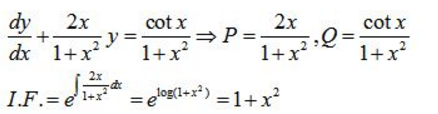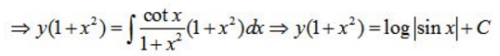# Test: Differential Equations (CBSE Level) - 2

## 25 Questions MCQ Test Mathematics (Maths) Class 12 | Test: Differential Equations (CBSE Level) - 2

Description
This mock test of Test: Differential Equations (CBSE Level) - 2 for JEE helps you for every JEE entrance exam. This contains 25 Multiple Choice Questions for JEE Test: Differential Equations (CBSE Level) - 2 (mcq) to study with solutions a complete question bank. The solved questions answers in this Test: Differential Equations (CBSE Level) - 2 quiz give you a good mix of easy questions and tough questions. JEE students definitely take this Test: Differential Equations (CBSE Level) - 2 exercise for a better result in the exam. You can find other Test: Differential Equations (CBSE Level) - 2 extra questions, long questions & short questions for JEE on EduRev as well by searching above.
QUESTION: 1

Solution:

2

QUESTION: 2

### General solution of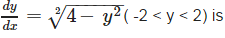Solution: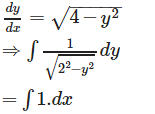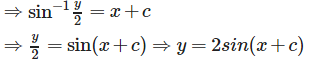QUESTION: 3

### In a bank, principal increases continuously at the rate of 5% per year. An amount of Rs1000 is deposited with this bank, how much will it worth after 10 years (e0.5= 1.648).

Solution: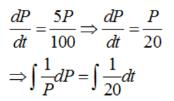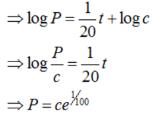When P = 1000 and t = 0 ., then ,
c = 1000, therefore, we have :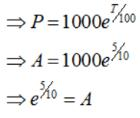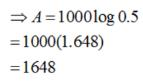QUESTION: 4

At any point (x, y) of a curve, the slope of the tangent is twice the slope of the line segment joining the point of contact to the point (– 4, –3). Find the equation of the curve given that it passes through (–2, 1).

Solution:

Slope of the line segment joining the point of contact P (x , y) to the point (- 4 , - 3) =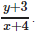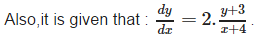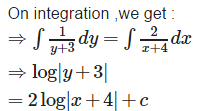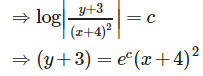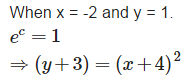QUESTION: 5

Solution of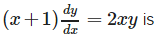Solution: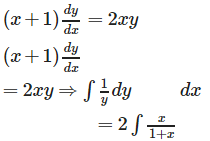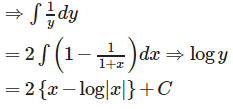QUESTION: 6

Find the order and degree of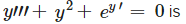Solution:

Order = 3 , degree not defined ,because the function y’ present in exponential form.

QUESTION: 7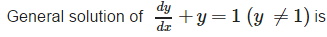Solution: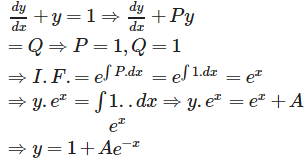QUESTION: 8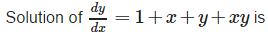Solution: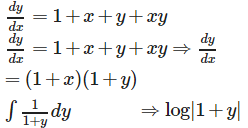QUESTION: 9

Solution of x dy− ydx =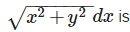Solution: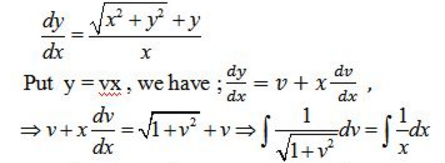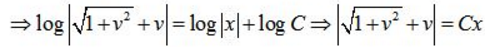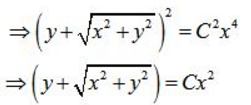QUESTION: 10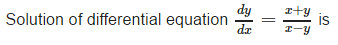Solution: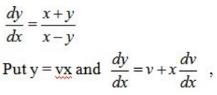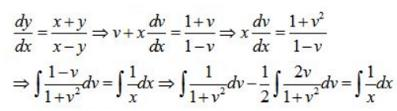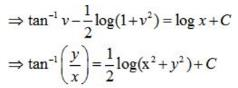QUESTION: 11

Determine order and degree (if defined) of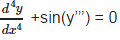Solution:

Order = 4 , degree not defined , because the function y’’’ present in the angle of sine function.

QUESTION: 12

General solution of sec2 x tany dx + sec2y tan x dy = 0 is

Solution: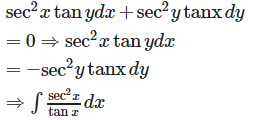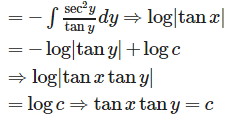QUESTION: 13

Find the particular solution for (x + y) dy + (x –y) dx = 0; y = 1 when x = 1

Solution: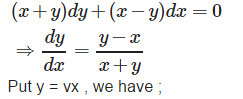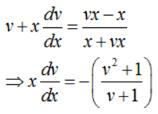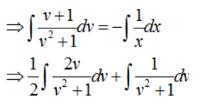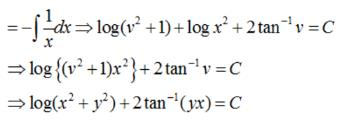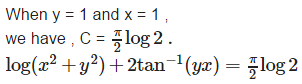QUESTION: 14

Variable separation method can be used to solve First Order, First Degree Differential Equations in which y’ is of the form.

Solution:

Variable separation method can be used to solveFirst Order, First Degree Differential Equations in which y’ is of the form. y’ = h(x)g(y) i.e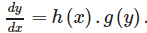QUESTION: 15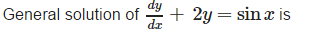Solution: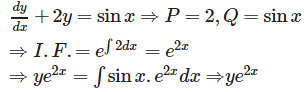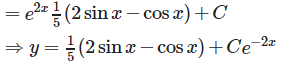QUESTION: 16

Determine order and degree (if defined) of y’ + 5y = 0

Solution:

Order = 1 , degree = 1.

QUESTION: 17

General Solution of (ex + e-x) dy - (ex - e-x) dx = 0

Solution: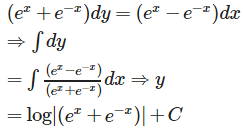QUESTION: 18

General solution of x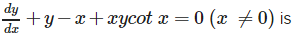Solution: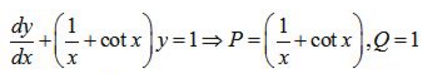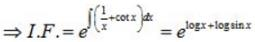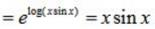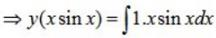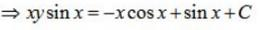QUESTION: 19

solution of {x cos (x/y) + ysin(y/x)} ydx = {ysin(y/x) - x cos (y/x)} xdy is

Solution: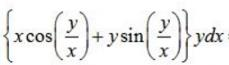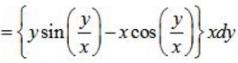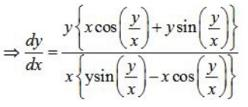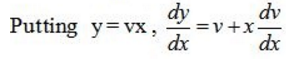QUESTION: 20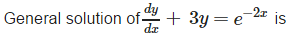Solution: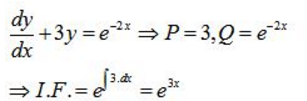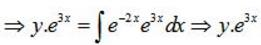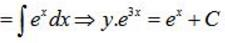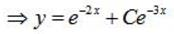QUESTION: 21

Determine order and degree (if defined) of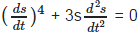Solution:

Order = 2 , degree = 1.

QUESTION: 22

General solution of y log y dx – x dy = 0

Solution: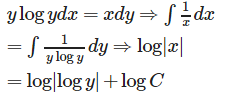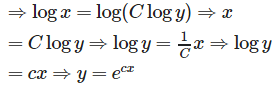QUESTION: 23

To form a differential equation from a given function

Solution:

To form a differential equation from a given functionDifferentiate thefunction successively as many times as the number of arbitrary constants inthe given function and eliminate the arbitrary constants.i.e. the differential equation should be free from arbitrary constants.

QUESTION: 24

A differential equation of the form y' = F(x,y) is homogeneous if

Solution:

A differential equation of the form y' = F(x,y) is homogeneous if F(x,y) is a homogeneous function of degree zero.

QUESTION: 25

General solution of(1+x2) dy+2xy dx = cot x dx(x ≠ 0) is

Solution: